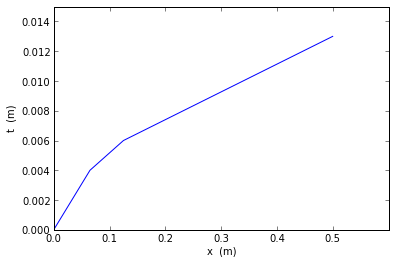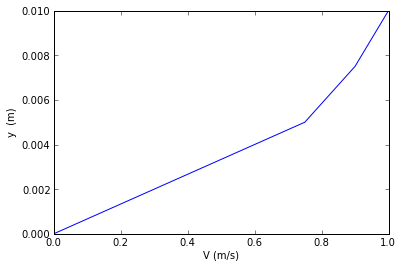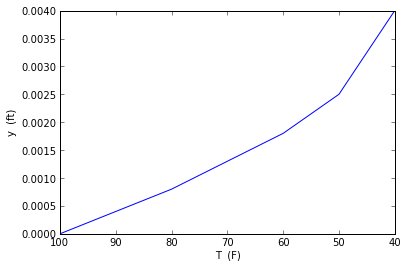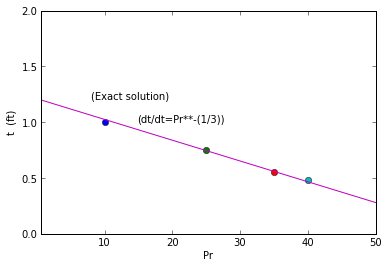# Chapter7 : Convection Heat Transfer in Flow Past immersed Bodies¶

### Example 7.1 page NO.353¶

In [ ]:
%matplotlib inline

In :
rou=1.177   # density in kg/cu.m
v=15.68e-6  # viscosity in sq.m/s
L=0.5       # length in m
V_inf=1;    # air velocity in m/s
Re= (V_inf*L)/v # Reynolds Number

import matplotlib.pyplot as plt
fig = plt.figure()

x=[0,0.065,0.125,0.5]
t=[0,0.004,0.006,0.013]
xlabel("x  (m)")
ylabel("t  (m)")
plt.xlim((0,0.6))
plt.ylim((0,0.015))
a1=plot(x,t)

import matplotlib.pyplot as plt
fig = plt.figure()

x=[0,0.75,0.9,1]
t=[0,0.005,0.0075,0.010]
xlabel("V (m/s)")
ylabel("y  (m)")
plt.xlim((0,1))
plt.ylim((0,0.010))
a1=plot(x,t)

gc=1
mu=rou*v/gc
b=1         # width in m
Df=0.664*V_inf*mu*b*(Re)**0.5

print"(a)plot between boundary layer growth with distance"
print"(b)velocity distribution with distance"
print"(c)The skin-drag including both sides of plate is ",round(2*Df,4),"n"

(a)plot between boundary layer growth with distance
(b)velocity distribution with distance
(c)The skin-drag including both sides of plate is  0.0044 n### Example 7.2 page NO.360¶

In [ ]:
%matplotlib inline

In :
rou= 62.4              #  density in Ibm/ft^3
cp=0.9988             # specific heat BTU/(lbm-degree Rankine)
v= 1.083e-5            # viscosity in sq.ft/s
kf = 0.345             # thermal conductivity in BTU/(hr.ft.degree Rankine)
a = 5.54e-3            # diffusivity in sq.ft/hr
Pr = 7.02             # Prandtl Number
V=1.2                 # velocity in ft/s

x1=1
Re1=(V*x1)/v   # Reynolds Number at x=1 ft
hL1=0.664*Pr**(1/3.0)*Re1**0.5*kf/x1
Tw=100           # temperature of metal plate in degree fahrenheit
T_inf=40           # temperature of water in degree fahrenheit
A1=x1*18/12.0 # cross sectional area for 1 ft length
q1=hL1*A1*(Tw-T_inf)

print"The heat transferred to water over the plate is",round(q1,0),"BTU/hr"
x2=2
Re2=(V*x2)/v   # Reynolds Number at x=1 ft
hL2=0.664*Pr**(1/3.0)*Re2**0.5*kf/x2
Tw=100           # temperature of metal plate in degree fahrenheit
T_inf=40           # temperature of water in degree fahrenheit
A2=x2*18/12.0 # cross sectional area for 1 ft length
q2=hL2*A2*(Tw-T_inf)
print"The heat transferred to water over the plate is ",round(q2,0),"BTU/hr"

import matplotlib.pyplot as plt
fig = plt.figure()

T=[100,80,60,50,40]
y=[0,0.0008,0.0018,0.0025,0.004]
xlabel("T  (F)")
ylabel("y  (ft)  ")
plt.xlim((100,40))
plt.ylim((0,0.004))
a1=plot(T,y)

import matplotlib.pyplot as plt
fig = plt.figure()

Pr=[0.6,50]
t=[1.2,0.28]
xlabel("Pr")
ylabel("t  (ft)  ")
plt.xlim((0.6,50))
plt.ylim((0,2))
ax.plot(, , 'o')
ax.annotate('(Exact solution)', xy=(8,1.2))
ax.annotate('(dt/dt=Pr**-(1/3))', xy=(15,1))
ax.plot(, [0.75], 'o')
ax.plot(, [0.55], 'o')
ax.plot(, [0.48], 'o')

a1=plot(Pr,t)

The heat transferred to water over the plate is 13141.0 BTU/hr
The heat transferred to water over the plate is  18584.0 BTU/hr### Example 7.3 page NO.363¶

In :
rou=0.998	 # density in kg/cu.m
cp=1009 		# specific heat in J/(kg*K)
v=20.76e-6 	# viscosity in sq.m/s
Pr=0.697 	# Prandtl Number
k=0.03003 	# thermal conductivity in W/(m.K)
a=0.2983e-4 	# diffusivity in sq.m/s
L=1.0 		  # Length of plate in m
V=5.0 		  # velocity of air in m/s
b=0.5 		# width in m
Re=V*L/v 	# Reynolds number at plate end

h=k*0.664*Re**(0.5)*Pr**(1/3.0)/L	     # The average convection coefficient in W/(sq.m.K)
Df=0.664*V*rou*v*b*(Re)**0.5 	       # drag force in N
hx=(1/2.0)*h                              # local convective coefficient
delta=5*L/(Re)**0.5 # The boundary-layer thickness at plate end
delta_t=delta/(Pr)**(1/3.0)

print"The local convective coefficient is   ",round(hx,2),"W/(sq.m.K)"
print"The boundary-layer thickness at plate end is  ",round(delta*100,2),"cm"
print"The thermal-boundary-layer thickness is  ",round(delta_t*100,2),"cm"

The local convective coefficient is    4.34 W/(sq.m.K)
The boundary-layer thickness at plate end is   1.02 cm
The thermal-boundary-layer thickness is   1.15 cm


### Example 7.4 page NO. 364¶

In :
rou= 0.0812             # density in Ibm/ft^3
cp=0.2918              # specific heat BTU/(lbm-degree Rankine)
v= 17.07e-5                # viscosity in ft^2/s
kf = 0.01546                 # thermal conductivity in BTU/(hr.ft.degree Rankine)
a = 0.8862              # diffusivity in ft^2/hr
Pr = 0.709               # Prandtl Number

qw=10/(1.5*10.125)*(1/.2918)*144  # The wall flux
V_inf=20               # velocity in ft/s
L=1.5/12               #  length in ft
Re_L=V_inf*10*L/v      # Reynolds number at plate end
T_inf=300                # free stream temperature in degree Rankine
Tw=T_inf+(qw*L*10/(kf*0.453*(Re_L)**0.5*(Pr)**(1/3.0)))

print"The maximum heater surface temperature is ",round(Tw,0),"R"

The maximum heater surface temperature is  470.0 R


### Example 7.5 page NO. 368¶

In :
rou= 0.0812            # density in Ibm/ft**3
cp=0.2918             # specific heat BTU/(lbm-degree Rankine)
v= 17.07e-5           # viscosity in ft**2/s
kf = 0.01546          # thermal conductivity in BTU/(hr.ft.degree Rankine)
a = 0.8862             # diffusivity in ft**2/hr
Pr = 0.709             # Prandtl Number
Tw=469                 # maximum heater temperature in degree Rankine
T_inf=300.0               # free-stream temperature in degree Rankine
qw=324.0                  # The wall flux in BTU/(hr.ft**2)
V_inf=20                # velocity in ft/s

hx=qw/(Tw-T_inf)        #  The convection coefficient
LHS=(hx/3600.0)*(Pr)**(2/3.0)/(rou*cp*V_inf)
Re_L=1.46e+005           # Reynolds number at plate end
RHS=0.332*(Re_L)**(-0.5)
err=(LHS-RHS)*100/LHS

print"The convection coefficient is  BTU/(hr.sq.ft.degree R)",hx
print"The error is ",round(err,0),"percent"
print"Since the error is only 3 percent, the agreement is quite good"

The convection coefficient is  BTU/(hr.sq.ft.degree R) 1.91715976331
The error is  3.0 percent
Since the error is only 3 percent, the agreement is quite good


### Example 7.6 page NO. 371¶

In :
rou= 62.4                    # density in Ibm/cu.ft
v= 1.083e-5                  # viscosity in sq.ft/s
V_inf=5*.5144/.3048          # barge velocity in ft/s using conversion factors from appendix table A1
L=20                         # Length of barge in ft
Re_L=V_inf*L/v               # Reynolds number at plate end
Cd=0.003                     #value of Coefficient of discharge figure 7.11
gc=32.2
b=12                          # width in ft

Df=(Cd*rou*V_inf**2*b*L)/(2*gc)

print"The drag force is ",round(Df,0),"lbf"

The drag force is  50.0 lbf


### Example 7.7 page NO. 374¶

In :
rou= 1.0732              # density in kg/m**3
cp= 1013                 # specific heat in J/(kg*K)
v= 21.67e-6              # viscosity in m**2/s
Pr = 0.702               # Prandtl Number
k= 0.03352               # thermal conductivity in W/(m.K)
a = 0.3084e-4            # diffusivity in m**2/s
V_inf=60                 # carbon dioxide velocity in m/s
vel=60                   #velocity

x_cr=(5e5)*v/V_inf       # The transition length in m
w=4.0                    # width of each heater in cm
b=0.16                    # effective heating length in m
Tw=600                   # temperature of heater surface in K
T_inf=400                # temperature of carbon dioxide in K

y=0.664*Pr**(1/3.0)*k*(vel/v)**(1/2.0)       #y=h1*x1**(1/2)
x1=0.04                  # m
h1=y/x1**(0.5)
q1=h1*(Tw-T_inf)*x1*b

x2=0.08
h2=y/(x2)**(0.5)
Q=h2*x2*(b)*(Tw-T_inf)      #Q=q1+q2
q2=Q-q1

x3=0.12
h3=y/(x3)**(0.5)
Q1=h3*x3*(b)*(Tw-T_inf)      #Q=q1+q2+q3
q3=Q1-Q

x4=0.16
h4=y/(x4)**(0.5)
Q2=h4*x4*(b)*(Tw-T_inf)      #Q=q1+q2+q3+q4
q4=Q2-Q1

Re5=vel*5*x1/(v)

h5=0.0359*(Re5**(0.8)-830)*Pr**(1/3.0)*(k/(11.9*x1))
x5=0.2
Q3=h5*x5*(b)*(Tw-T_inf)      #Q3=q1+q2+q3+q4+q5
q5=Q3-Q2

Re6=vel*6*x1/(v)
h6=0.0359*(Re5**(0.8)-830)*Pr**(1/3.0)*(k/(10.3*x1))
x6=0.24
Q4=h6*x6*(b)*(Tw-T_inf)      #Q4=q1+q2+q3+q4+q5+q6
q6=round(Q4,0)-round(Q3,0)

print"The wattage required for 1st heater is ",round(q1,0),"W"
print"The wattage required for 2nd heater is ",round(q2,0),"W"
print"The wattage required for 3rd heater is ",round(q3,1),"W"
print"The wattage required for 4th heater is ",round(q4,0),"W"
print"The wattage required for 5th heater is ",round(q5,0),"W"
print"The wattage required for 6th heater is ",round(q6,1),"W"

The wattage required for 1st heater is  211.0 W
The wattage required for 2nd heater is  87.0 W
The wattage required for 3rd heater is  67.0 W
The wattage required for 4th heater is  56.0 W
The wattage required for 5th heater is  132.0 W
The wattage required for 6th heater is  213.0 W


### Example 7.8 page NO. 302¶

In :
rou= 0.0735          # density in Ibm/ft**3
v= 16.88e-5          # viscosity in ft**2/s
V=20*5280/3600.0        # flow velocity in ft/s
D=1.0               # diameter of pole in ft
L=30.0                 # length of pole in ft
gc=32.2
Re_D=V*D/v            # Reynolds Number for flow past the pole
Cd_cylinder=1.1       # value of Cd for smooth cylinder from figure 7.22
A_cylinder=D*L         #  frontal area of pole

Df_cylinder=Cd_cylinder*(0.5)*rou*V**2*A_cylinder/gc
D_square=2/12.0          # length of square part of pole
L_square=4
Re_square=V*D_square/v # Reynolds Number for square part of pole
Cd_square=2            # Corresponding value of Cd for square part from figure 7.23
A_square=D_square*L_square      # projected frontal area of square part
Df_square=Cd_square*(0.5)*rou*V**2*A_square/gc
Df_total=Df_cylinder+Df_square
print"The total drag force on the pole is ",round(Df_total,1),"lbf"

The total drag force on the pole is  33.7 lbf


### Example 7.9 page NO. 387¶

In :
rou= 0.883        # density in kg/cu.m
cp= 1014          # specific heat in J/(kg*K)
v= 25.90e-6       # viscosity in sq.m/s
Pr = 0.689        # Prandtl Number
kf= 0.03365       # thermal conductivity in W/(m.K)
a = 0.376e-4      # diffusivity in sq.m/s
V_inf=1           # velocity in m/s
D=0.00013         # diameter in m
L=0.01           # length of wire in cm
Re_D=V_inf*D/v    # The Reynolds number of flow past the wire
C=0.911           #value of C for cylinder from table 7.4
m=0.385           #value of m for cylinder from table 7.4

hc=kf*C*(Re_D)**m*(Pr)**(1/3)/D   # the convection coefficient in W/(m**2.K)
Tw=500 # air stream temperature in K
T_inf=300 # wire surface temperature in K
As=math.pi*D*L # cross sectional area in sq.m
qw=hc*As*(Tw-T_inf) # The heat transferred to the air from the wire
resistivity=17e-6 # resistivity in ohm cm
Resistance=resistivity*(L/(math.pi*D**2)) # resistance in ohm
i=(qw*100/Resistance)**0.5 # current in ampere

print"The current is %.1f A",round(i,1),"A"

The current is %.1f A 3.3 A


### Example 7.10 page NO.393¶

In :
rou= 0.0735          # density in Ibm/cu.ft
cp=0.240            # specific heat BTU/(lbm-degree Rankine)
v= 16.88e-5         # viscosity in sq.ft/s
kf = 0.01516        # thermal conductivity in BTU/(hr.ft.degree Rankine)
a = 0.859           # diffusivity in sq.ft/hr
Pr = 0.708          # Prandtl Number
OD=0.875/12        # outer diameter in ft
ID=0.06208          # inner diameter in ft
A=0.003027         # cross sectional area in sq.ft
L=2
sL=1.5/12
sT=1.3/12
V_inf=12           # velocity of air in ft/s

V1=(sT*V_inf)/(sT-OD)         # velocity at area A1 in ft/s
sD=((sL)**2+(sT/2)**2)**0.5   # diagonal pitch in inch
V2=(sT*V_inf)/(2*(sD-OD))
Vmax=V1
Re_D=Vmax*OD/v     # Reynolds Number
sT_OD=1.3/0.875
sT_sL=1.3/1.5
f1=0.35            #  value of f1 for above values of sT/Do and Re
f2=1.05            #Corresponding value of f2 for above values of sT/sL and Re
gc=32.2
N=7
dP=N*f1*f2*(rou*Vmax**2/(2*gc))
sL_Do=sL/OD
C1=0.438           #value of C1 for above values of sT/Do and sL/Do
C2=0.97            #value of C2 for above values of sT/Do and sL/Do
m=0.565            #value of m for above values of sT/Do and sL/Do
hc=kf*1.13*C1*C2*(Re_D)**m*(Pr)**(1/3.0)/OD # The convection coefficient
As=70*math.pi*OD*L # outside surface area of 70 tubes
Tw=200              # outside surface temeperature in degree F
T_inf=70            #  air temperature in degree F
q=hc*As*(Tw-T_inf)  # heat transferred

print"The pressure drop is  ",round(dP/147,3),"psi"
print"The heat transferred is",round(q,1)," BTU/hr"

The pressure drop is   0.027 psi
The heat transferred is 87571.6  BTU/hr

In [ ]: